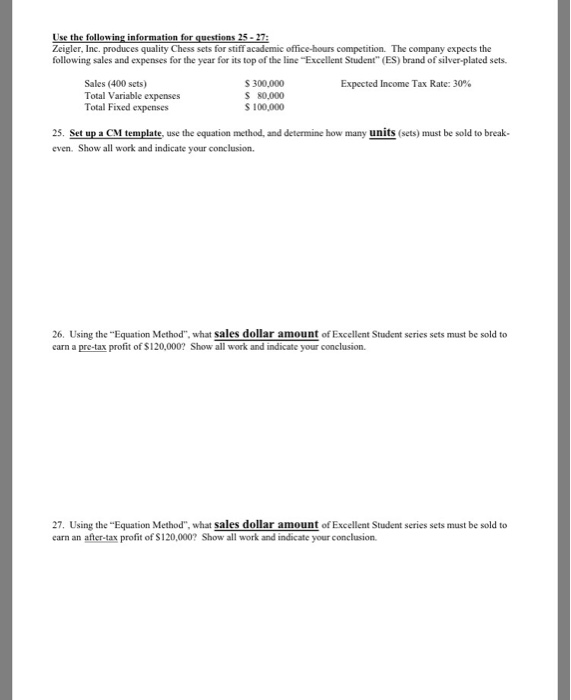# Question & Answer: Use the following information for questions 25 – 27: Zeigler. Inc. produces quality Chess sets…..Use the following information for questions 25 – 27: Zeigler. Inc. produces quality Chess sets for stiff academic office-hours competition. The company expects the following sales and expenses for the sear for its top of the line “Excellent Student” (ES) brand of silver-plated sets. Sales (400 sets) \$ 300,000 Expected income Tax Rate: 30% Total Variable expenses \$ 80,000 Total Fixed expenses \$100,000 25. Set up a CM template, use the equation method, and determine how mans units (sets) must be sold to break-even and indicate your conclusion. 26. Using the “Equation Method”, what Sales dollar amount of Excellent Student series sets must be sold to earn a pre-tax profit of \$120,000? and indicate your conclusion. 27. Using the “Equation Method”, what sales dollar amount of Excellent Student series sets must be sold to cam an after-tax profit of SI20.000? Show- all work and indicate your conclusion.

Don't use plagiarized sources. Get Your Custom Essay on
Question & Answer: Use the following information for questions 25 – 27: Zeigler. Inc. produces quality Chess sets…..
GET AN ESSAY WRITTEN FOR YOU FROM AS LOW AS \$13/PAGE

As per Equation Method:

Profit= Sales – Variable Expenses- Fixed Expense
At Break Even Point, Profit is Zero

Selling Price per unit = 300,000 / 400 = \$750
Variable Expenses per unit = 80,000 / 400 = \$200

Profit= Sales – Variable Expenses- Fixed Expense
Let the units be sold be “X” units
\$0 = (\$750 * x) – (\$200 * x) – \$100,000
\$0 = \$550x – \$100,000
x = 181.81 or 182 units

As per Equation Method:

Profit= Sales – Variable Expenses- Fixed Expense

Selling Price per unit = 300,000 / 400 = \$750
Variable Expenses per unit = 80,000 / 400 = \$200

Profit= Sales – Variable Expenses- Fixed Expense
Let the units be sold be “X” units
\$120,000 = (\$750 * x) – (\$200 * x) – \$100,000
\$120,000 = \$550x – \$100,000
x = 400 units

Therefore, 400 units must be sold to earn a Pre-tax profit of \$120,000.

As per Equation Method:

After- tax Profit= (Sales – Variable Expenses- Fixed Expense)* (1- Tax rate)

Selling Price per unit = 300,000 / 400 = \$750
Variable Expenses per unit = 80,000 / 400 = \$200

After- tax Profit= (Sales – Variable Expenses- Fixed Expense)* (1- Tax rate)

Let the units be sold be “X” units
\$120,000 = [(\$750 * x) – (\$200 * x) – \$100,000] * (1 – 0.30)
\$120,000 = (\$550x – \$100,000) * 0.70
\$120,000 = \$385x – \$70,000
\$190,000 = \$385x
x = 493.51 unit or 494 units

Therefore, 494 units must be sold to earn after-tax profit of \$120,000.